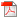# Vertical　Production Chain, Trade and Tariffs　in a Two-Factor Model

### 要約

This paper investigates the Heckscher-Ohlin theorem, the Rybczynski theorem, and the Stolper-Samuelson theorem in a two-factor vertical production chain model of trade. For this purpose, capital is introduced into the model of Yano and Dei (2003). A primary analytic device is the economy-wide production curve. This curve is derived under autarky, free trade, and tariffs, respectively.(_FILE_SIZE_)